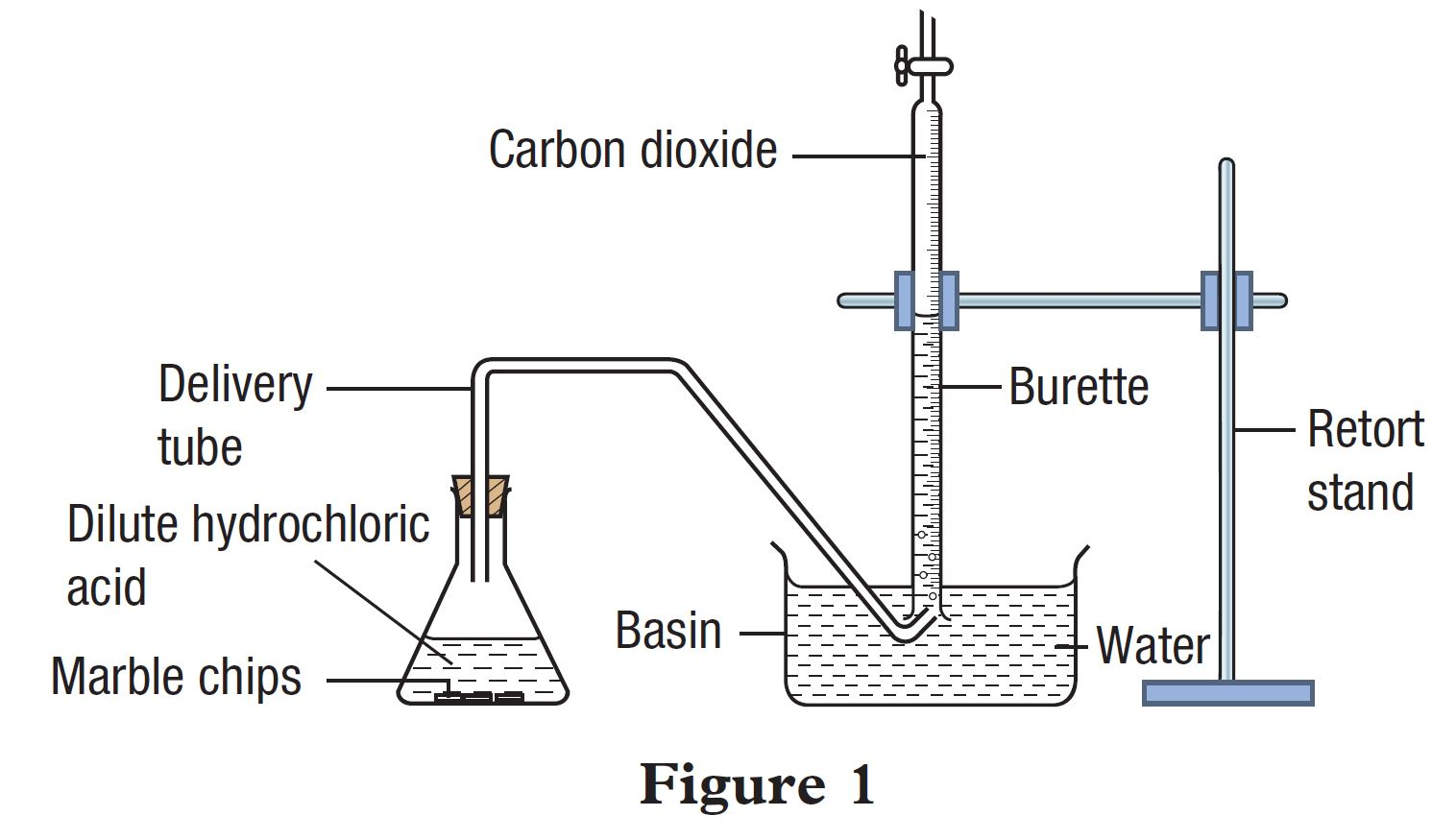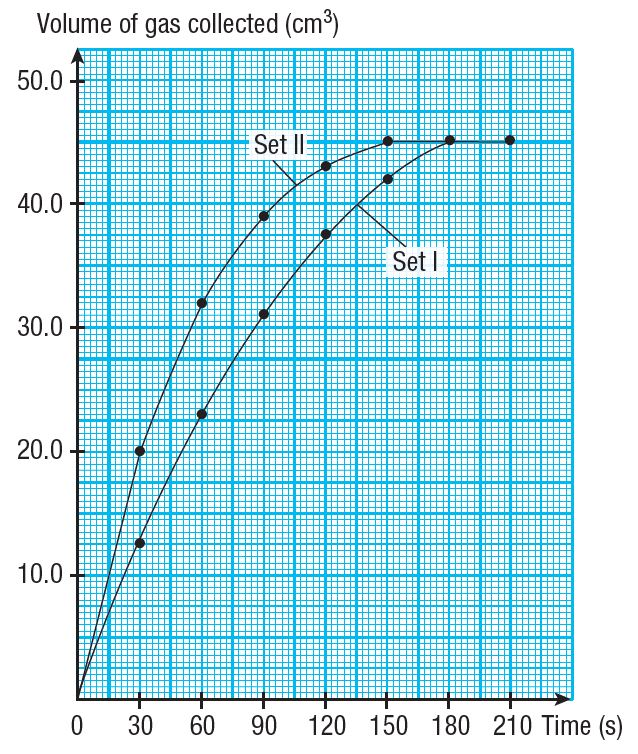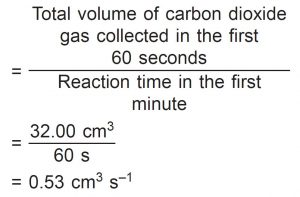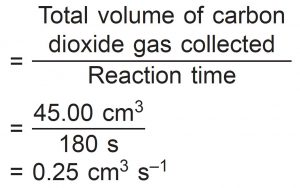# Summative Practice (Question 1 & 2) – Form 5 Science (KSSM) Chapter 4

Question 1:
(a) What is meant by chemical reaction?
(b) Is the rate of reaction affected by pressure? Explain your answer.

(a)
Process where one or more reactants are converted into one or more products.

(b)
Yes.
Pressure affects the reaction for reactants in the gaseous state.

Question 2:
A student carried out an experiment to study a factor which affects the rate of reaction between marble (calcium carbonate) and dilute hydrochloric acid. Figure 1 shows the apparatus set-up for the experiment.The student carried out the experiment using marble chips (Set I) and repeated the experiment by replacing the marble chips with marble powder (Set II). Table 1 shows the results of the experiment for Set I and Set II.(a) In this experiment, state the:
(i) manipulated variable
(ii) responding variable
(iii) constant variable

(b) State one hypothesis for this experiment.

(c) Based on Table 1, draw two graphs of volume of gas collected against time for Set I and Set II experiments on the same set of axis on a graph paper.

(d) Based on Set II, calculate:
(i) average rate of reaction for the first minute
(ii) average rate of reaction for the first two minutes
(iii) average rate of reaction in the second minute
(iv) rate of reaction at the 60th second
(v) average rate of reaction for the whole reaction

(e) Based on the results of Set I, calculate the average rate of reaction for the whole reaction.

(a)(i) Size of marble chips/calcium carbonate

(a)(ii) Volume of gas collected

(a)(iii)
Concentration of hydrochloric acid, volume of hydrochloric acid and mass of marble chips

(b)
The smaller the size of marble chips/ calcium carbonate, the higher the rate of reaction.

(c)(d)(i)
Average rate of reaction in the first minute(d)(ii)
Average rate of reaction in the first two minutes(d)(iii)
Average rate of reaction in the second minute(d)(iv)
Rate of reaction at the 60th second(d)(v)
Average rate of reaction for the whole reaction(e)
Average rate of reaction for the whole reaction